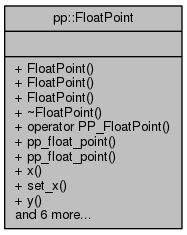Pepper_31_C++_interfaces
pp::FloatPoint Class Reference

`#include <point.h>`

Collaboration diagram for pp::FloatPoint:## Public Member Functions

FloatPoint ()
A constructor for a point at 0,0. More...

FloatPoint (float in_x, float in_y)

FloatPoint (const PP_FloatPoint &point)

~FloatPoint ()
Destructor. More...

operator PP_FloatPoint () const

const PP_FloatPoint & pp_float_point () const

PP_FloatPoint & pp_float_point ()

float x () const

void set_x (float in_x)

float y () const

void set_y (float in_y)

FloatPoint operator+ (const FloatPoint &other) const

FloatPoint operator- (const FloatPoint &other) const

FloatPointoperator+= (const FloatPoint &other)

FloatPointoperator-= (const FloatPoint &other)

void swap (FloatPoint &other)

## Detailed Description

A 2 dimensional floating-point point with 0,0 being the upper-left starting coordinate.

Definition at line 155 of file point.h.

## Constructor & Destructor Documentation

 pp::FloatPoint::FloatPoint ( )
inline

A constructor for a point at 0,0.

Definition at line 158 of file point.h.

Referenced by operator+(), and operator-().

 pp::FloatPoint::FloatPoint ( float in_x, float in_y )
inline

A constructor accepting two values for x and y and converting them to a FloatPoint.

Parameters
 [in] in_x An value representing a horizontal coordinate of a point, starting with 0 as the left-most coordinate. [in] in_y An value representing a vertical coordinate of a point, starting with 0 as the top-most coordinate.

Definition at line 171 of file point.h.

 pp::FloatPoint::FloatPoint ( const PP_FloatPoint & point )
inline

A constructor accepting a pointer to a PP_FloatPoint and converting the PP_Point to a Point. This is an implicit conversion constructor.

Parameters
 [in] point A PP_FloatPoint.

Definition at line 180 of file point.h.

 pp::FloatPoint::~FloatPoint ( )
inline

Destructor.

Definition at line 185 of file point.h.

## Member Function Documentation

 pp::FloatPoint::operator PP_FloatPoint ( ) const
inline

A function allowing implicit conversion of a FloatPoint to a PP_FloatPoint.

Definition at line 190 of file point.h.

 FloatPoint pp::FloatPoint::operator+ ( const FloatPoint & other ) const
inline

Adds two Points (this and other) together by adding their x values and y values.

Parameters
 [in] other A Point.
Returns
A new Point containing the result.

Definition at line 238 of file point.h.

References FloatPoint(), x(), and y().

 FloatPoint& pp::FloatPoint::operator+= ( const FloatPoint & other )
inline

Adds two Points (this and other) together by adding their x and y values. Returns this point as the result.

Parameters
 [in] other A Point.
Returns
This Point containing the result.

Definition at line 258 of file point.h.

References x(), and y().

 FloatPoint pp::FloatPoint::operator- ( const FloatPoint & other ) const
inline

Subtracts one Point from another Point by subtracting their x values and y values. Returns a new point with the result.

Parameters
 [in] other A FloatPoint.
Returns
A new Point containing the result.

Definition at line 248 of file point.h.

References FloatPoint(), x(), and y().

 FloatPoint& pp::FloatPoint::operator-= ( const FloatPoint & other )
inline

Subtracts one Point from another Point by subtracting their x values and y values. Returns this point as the result.

Parameters
 [in] other A Point.
Returns
This Point containing the result.

Definition at line 270 of file point.h.

References x(), and y().

 const PP_FloatPoint& pp::FloatPoint::pp_float_point ( ) const
inline

Getter function for returning the internal PP_FloatPoint struct.

Returns
A const reference to the internal PP_FloatPoint struct.

Definition at line 197 of file point.h.

 PP_FloatPoint& pp::FloatPoint::pp_float_point ( )
inline

Getter function for returning the internal PP_Point struct.

Returns
A mutable reference to the PP_Point struct.

Definition at line 204 of file point.h.

 void pp::FloatPoint::set_x ( float in_x )
inline

Setter function for setting the value of x.

Parameters
 [in] in_x A new x value.

Definition at line 216 of file point.h.

 void pp::FloatPoint::set_y ( float in_y )
inline

Setter function for setting the value of y.

Parameters
 [in] in_y A new y value.

Definition at line 228 of file point.h.

 void pp::FloatPoint::swap ( FloatPoint & other )
inline

Swaps the coordinates of two Points.

Parameters
 [in] other A Point.

Definition at line 279 of file point.h.

References x(), and y().

 float pp::FloatPoint::x ( ) const
inline

Getter function for returning the value of x.

Returns
The value of x for this Point.

Definition at line 211 of file point.h.

Referenced by operator+(), operator+=(), operator-(), operator-=(), operator==(), and swap().

 float pp::FloatPoint::y ( ) const
inline

Getter function for returning the value of y.

Returns
The value of y for this Point.

Definition at line 223 of file point.h.

Referenced by operator+(), operator+=(), operator-(), operator-=(), operator==(), and swap().

The documentation for this class was generated from the following file: